ClusTree: The Hierarchical Clustering Analyzer (Version 1.0)

### Outline

ClusTree is a GUI Matlab tool that:
• Enables an easy and intuitive way to cluster, analyze and compare some hierarchical clustering methods
• Consists of a two-step wizard that wraps some basic Matlab clustering methods and introduces the Top-Down Quantum Clustering algorithm.
• Provides a flexible and customizable interface for clustering data with high dimensionality.
• Allows both textual and graphical display for the clustering results.
• Can either apply hierarchical clustering to a dataset or analyze ‘pre-clustered’ results.

### How to install?

ClusTree is a self-extracting package. In order to install and run the ClusTree tool, please follow these three easy steps:

3. In Matlab,type 'clustree' at the command prompt.

### Starting ClusTree

After typing 'clustree' at the command prompt the main window will open.
1. ClusTree: Main window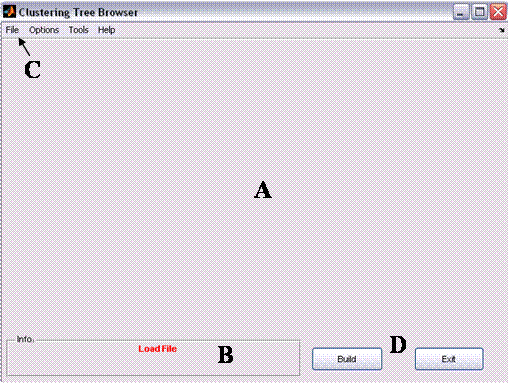Figure 1: Clustree's main window

The main window consists of four areas:

A. Graphical window - Area in which the graphical clustering result (i.e., the tree) is displayed. When no tree is loaded (e.g., prior the clustering) this area will be empty.
B. Information area - the area in which the status is displayed (when no data is loaded, a red ‘Load file’ indication is displayed). When a tree is loaded and the external classification is available (see Tree Options below) , three clustering scores are displayed (see ‎1.2.i below).
C. Menus Bar - the area in which the some actions are available: data loading, data processing, display options, detailed evaluation options etc.
D. Command Buttons - 2 main buttons: ‘Build’ - build a tree option (applicable only after clustering is applied) and ‘Exit’ - safely exiting the application.

### Main Workflow

#### 1. New clustering /Open ‘pre-clustered’ data

ClusTree can either cluster a given dataset or analyze a dataset that has already been clustered

##### i. New clustering - analyzing a 'raw' dataset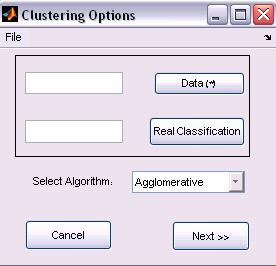Figure 2: New Clustering options

ClusTree receives three input parameters, which are Matlab variables (i.e., must be defined in Matlab workspace before running the ClusTree).

• Data (Mandatory field): two-dimensional matrix of doubles. Represents the elements (objects to be clustered are the matrix’s columns).
• Real classification (Optional field): one-dimensional vector. Represents the real classification of the elements (i.e., the class of the i-th element which appears in the i-th place in the vector). Please note that the vector’s length must be equal to the number of elements in the Data matrix (Otherwise, an error will occurr and will be flagged).
• The two input parameters can be typed in or selected from the base workspace.
• Clustering Algorithms (Agglomerative, TDQC, PDDP)
1. Agglomerative Clustering: The Bottom-Up Agglomerative clustering algorithm is the default option of the Matlab environment (See Matlab, Statistics Toolbox 5.1 manual). Please schoos the Data representation, Distance measure and Linkage type options.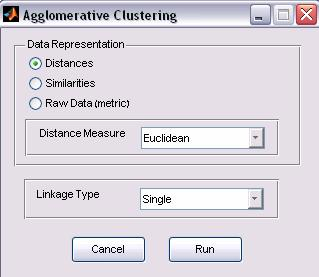Figure 3: Agglomerative Clustering options

a.     Data Representation: the input data matrix can be represented in various ways

• Distances: a symmetric squared matrix in which the value in the ij place represents the distance between elements i and j.
• Similarities: a symmetric squared matrix in which the value in the ij place represents the similarity between elements i and j.
• Raw Data: a features-space matrix, which the value in the ij place represents the value of feature j for element i.

The user should specify in which of the three options the data is represented. If the data representation is either Distances or Similarity, the Distance Measure option is disabled and ignored. Otherwise (Raw data), the user should select one of the Distance Measure options.
b.     Distance Measure

• euclidean
• seuclidean
• cityblock
• mahalanobis
• minkowski
• cosine
• correlation
• hamming
• jaccard
• chebychev

c.      Linkage Type - After the distance is measure, a linkage is applied. The Linkage types are:

• single
• complete
• average
• weighted
• centroid
• median
• ward

2.     Top-Down-Quantum-Clustering (TDQC) Options: the options can be specified in a configuration file (advanced mode), or by setting the parameters.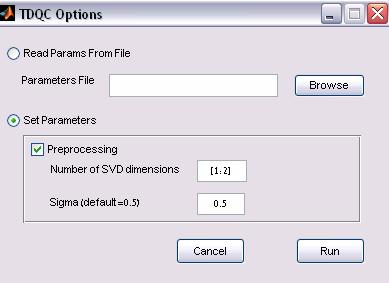Figure 4: TDQC Clustering options

a.     Parameter file option: The TDQC can be applied to a configuration input file. The input file format is as follows:

 % A line that starts with a ‘%’ is a comment % data=[m*n data matrix] * mandatory data=x  %realmapping=[1*n classification] optional realmapping=y %preprocessing= [0-no, 1 - SVD, 2 - SVD + normalization] preprocessing=2 % algorithm=4 --> must remain constant! algorithm=4 %dims=[start:end]--> dimensions dims=[1:2] %clustercolumns=1 --> must remain constant! clustercolumns=1 %steps=[for gradient descend] steps=50 %numelems=[ number of elements] numelems=200 %sigma=[for qc] sigma=0.5

b.     Direct input option

• Preprocessing – whether to cluster normalized, truncated SVD vectors (selected by default)
• Sigma value – the Parzen window size (default is 0.5).

3.     PDDP Clustering (when installed: the optional PDDP package should be downloaded separately from http://www-users.cs.umn.edu/~boley/PDDP.html)

Once the algorithm is chosen and applied, the status message in the information bar is changed to ‘loaded’

##### ii. Open previously saved results

Browsing previously saved file. The file is a Matlab data file (.mat) with two parameters: parent (required) and realClass (optional)

• parent: an (m-1)-by-3 matrix. The output of the Matlab ‘linkage’ function. parent is an containing cluster tree information. The leaf nodes in the cluster hierarchy are the objects in the original data set, numbered from 1 to m. They are the singleton clusters from which all higher clusters are built. Each newly formed cluster, corresponding to row i in parent, is assigned the index m+i, where m is the total number of initial leaves. Columns 1 and 2, parent (i,1:2), contain the indices of the objects that were linked in pairs to form a new cluster. This new cluster is assigned the index value m+i. There are m-1 higher clusters that correspond to the interior nodes of the hierarchical cluster tree. Column 3, parent (i,3), contains the corresponding linkage distances between the objects paired in the clusters at each row i.
• Note: ClusTree ignores the 3rd column (the linkage distances).
•  realClass (Optional field) - one-dimensional vector. Represents the real classification of the elements (i.e., the class of the i-th element appears in the i-th place in the vector). Please note that, the vector’s length must be equal to the number of elements in the Data matrix (Otherwise an error will occur).

#### 2. Build the tree

the "Build tree" option is applicable after data is clustered or a pre-clustered data is successfully loaded. Clicking the ‘Build’ button (area D) will construct the tree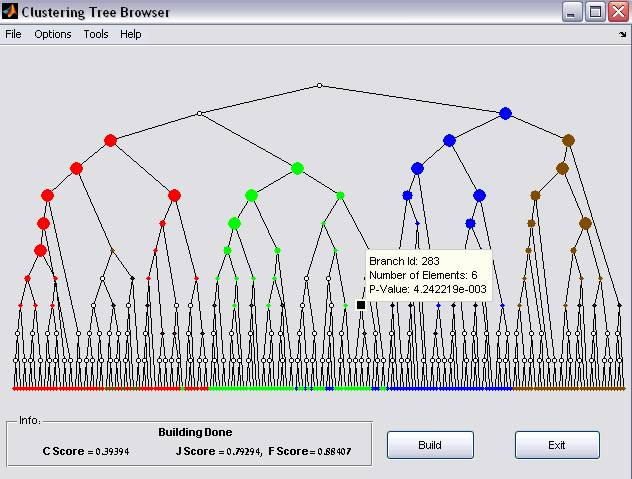Figure 5: Clustering results Dot sizes indicate statistical enrichment levels (larger sizes correspond to smaller p-values). Uncolored nodes represent non-significant enrichment

The clustering tree represents a parent-child relation in which the leaf nodes represent data-elements and tree branched represent a cluster (that includes the nodes below).

If the real classification information is available and paint level is set to branches (see ‎3 below), clustering evaluation can be presented. Since each node specifies a cluster, enrichment p-values can be calculated to assign the given node with one of the classes in the data. This is done by using the hypergeometric probability density function. The significance p-value of observing k elements assigned by the algorithm to a given category in a set of n elements is given by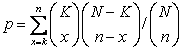, where K is the total number of elements assigned to the class (the category) and N is the number of elements in the dataset. The p-values for all nodes and all classes may be viewed as dependent set estimations; hence we apply the False Discovery Rate (FDR) criterion to them requiring q<0.05 . P-values which do not pass this criterion are considered non-significant. We further apply another conservative criterion; namely, a node is considered significant only if k≥n/2 (i.e., the majority of its elements belongs to the enriched category).

In the graphical results, Dot sizes indicate statistical enrichment levels (larger sizes correspond to smaller p-values). Uncolored nodes represent non-significant enrichment (for modifications of the above configurations see ‎3 below).

###### Branch information

By selecting a tree branch, a tooltip floating window appears (Figure 5). The tooltip displays the Branch id, number of elements it includes, and the significance enrichment p-values. The list of elements that belong to the selected branch can be exported using the export command (see ‘Export current’ below)

###### Scoring the Tree

1.      C Score – the relative number of significant branches (clusters) [#significant branches/ the # of branches in the tree]
2.      J Score We define the weighted best-J-Score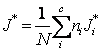,
where J*i is the best J-Score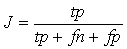where tp is the number of true positive cases, fn the number of false negative cases and fp the number of false positive cases), for class i in the tree, ni is the number of data-points (i.e., elements) in class i, c is the number of classes and N is the number of data-points in the dataset. This criterion provides a single number specifying the quality of the tree based on a few nodes that contain optimal clusters.

3.      F Score – similarly to the J Score, the weighted best-F-Score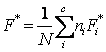where F*i is the best F-Score,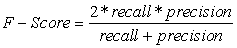where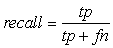,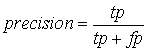for class i in the tree, ni is the number of data-points in class i, c is the number of classes and N is the number of data-points in the dataset

#### 3. Analyze the tree

In addition to the visualization and scoring options, ClusTree provides additional analysis tools (scores Distribution and Ultrametric Display)
i. Scores Distribution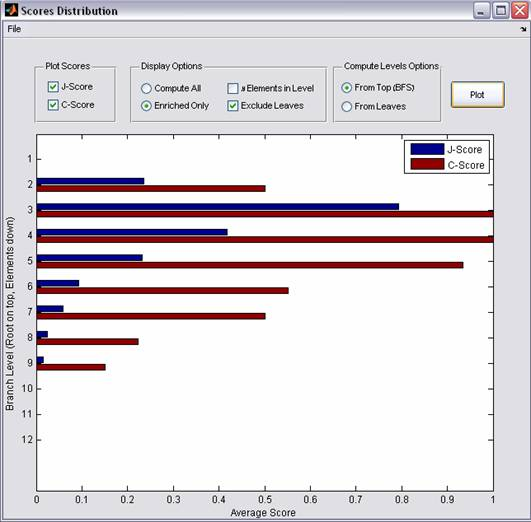Figure 6: Visualization Scores Distribution

The Scores Distribution displays the Levels scores. A level l of the tree contains all nodes that are separated by l edges from the root, i.e., that share the same Breadth First Search (BFS) mapping. Each level specifies a partition of the data into clusters. Choosing for each node, the class for which it turned out to have a significant node score, we evaluate its J-score (see above). If the node in question has been judged to be non-significant by the enrichment criterion, its J-score is set to null. The level score is defined as the average of all J-scores at the given level.
In addition to the above definitions, the Levels C scores can be displayed and some modifications can be applied (displaying the number of branches in each level, including non-significant branches and computing levels from leaf nodes instead of from the root, see Figure 6).

ii. Ultrametric Display

The resulting tree defines an Ultrametric dimension, where the distance between two leaf-nodes is defined as the number of edges connecting them.
The Ultrametric information is graphically displayed and can be exported in a textual format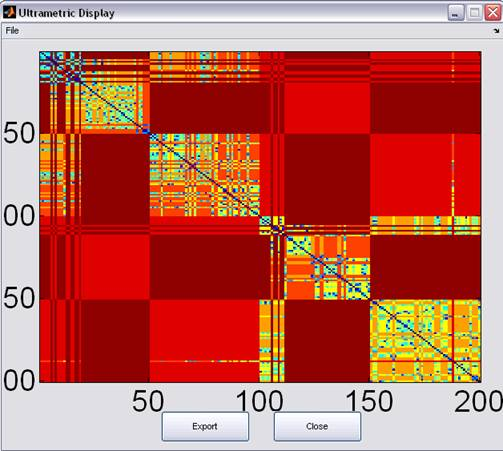Figure 7: Ultrametric Display.
• Export current branch: If a branch is selected (see Branch information above and Figure 5), its properties can be exported to either a set of Matlab variables or to a text file.
• Print graphical information – printing the graphical results of the clustering (i.e., the tree)
• Save current results – save the current environment for future analysis (output file can be served as an input file, see ‘Open previously saved results’ above)

#### 4. Visualization Options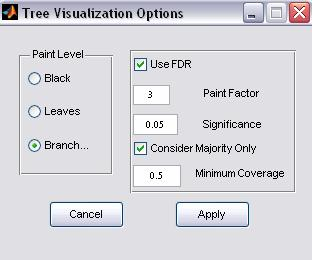Figure 8: Visualization options

ClusTree allows the configuration of the default visualization settings.

• Paint Level
• Black – regardless if the real classification is available or not, the tree is displayed as black (i.e., nodes and branches are not colored). If this option is selected all clustering evaluation information is not available.
• Leaves (applicable only if real classification is available) - leaf nodes are colored according to their classification association
• Branches (default) - (applicable only if real classification is available) – both leaf nodes and branches are colored (for more information, see ‎2 above).
• FDR (False Discovery Rate) Option: The p-values for all nodes and all classes may be viewed as dependent set estimations; hence we suggest applying the False Discovery Rate (FDR) criterion to them. Nevertheless, if user prefers not to apply FDR correction, the FDR option should be unselected.
• Paint Factor: the number indicates the relative size of the nodes size.  Note: This option is for visualization purposes only.
• Significance: the p-value threshold for specifying a significant branch
• Majority Consideration: if this option is checked, the user can specify a coverage threshold for coloring a branch (values range from 0 to 1). For example if the user specifies 0.5, A branch is considered significant only more than 50% of its elements belong to the enriched cluster. Please note: the value 1 means a ‘complete homogeneity’ (i.e., all the elements in every colored branch belong to the enriched cluster)

### Optional extensions

ClusTree is a set of self explanatory and documented Matlab functions and as such can be extended. Users who are familiar with Matlab and wish to change features in the current tool are welcome to do so. In addition, the tool was designed so that adding a new clustering method requires changing only one function.

### Requirements

1. Project name: ClusTree: The Hierarchical Clustering Analyzer
3. Operating system(s): Platform independent tested on MS-Windows (2000, XP), Linux and Unix
4. Programming language: Matlab
5. Other requirements: Matlab 7 or higher, Statistics Toolbox 5.1, COMPACT, and the PDDP package (optionally, should be downloaded separately from http://www-users.cs.umn.edu/~boley/PDDP.html)